# High Pass RC Circuit Response to a Square Wave

The study the response of high pass RC circuits to a square wave input; numerical examples with graphs of voltages are presented.
An online calculator and grapher on high pass RC circuit response to a square wave is also included.



## Problem with Solution

Find and graph the voltages across the capacitor $R$ as function of time in the high pass $RC$ ciruit below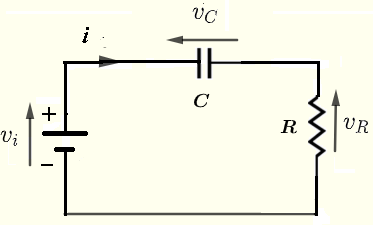Fig.1 - High Pass RC circuit given that the input voltage is $v_i(t)$ is a square wave as shown in the graph below.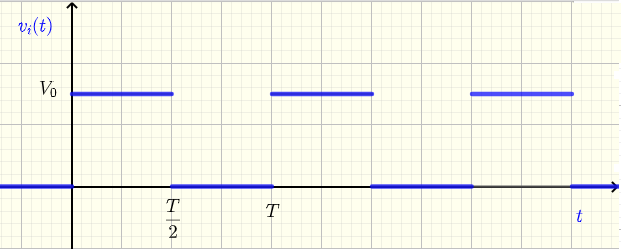Fig.2 - Square Wave as Input to the high pass RC Circuit Solution to the Problem
In the study of the low pass RC circuit response to a square wave, it was found that the voltage across the capacitor is given by
$\displaystyle v_C(t) = \displaystyle V_0 \sum_{n=0}^{n=\infty} \left \{ u(t-nT) \; \left(1 - e^{- \dfrac{t - n \; T}{R \;C} } \right) \\\\ \quad \quad \quad \quad - u(t-(n+1/2)T) \; \left(1 - e^{-\dfrac{ t - (n + 1/2) T}{\; R \; C} } \right) \right\}$
when the input $v_i(t)$ voltage is a square wave modelled by a sum of positive and negative step unit functions of the form

$\displaystyle v_i(t) = V_0 \sum_{n=0}^{n=\infty} \left\{ u(t - n\;T)- u (t-(n+1/2)\;T) \right\}$
In this study we need to find the voltage $v_R(t)$ cross the resistor which is given by
$v_R(t) = v_i(t) - v_C(t)$
When $v_i(t)$ and $v_C(t)$ are substituted by their expression given above, we can simplify $v_R(t)$ to
$\displaystyle v_R(t) = \displaystyle V_0 \sum_{n=0}^{n=\infty} \left \{ u(t-nT) \; \left(e^{- \dfrac{t - n \; T}{R \;C} } \right) \\\\ \quad \quad \quad - u(t-(n+1/2)T) \; \left(e^{-\dfrac{ t - (n + 1/2) T}{\; R \; C} } \right) \right\}$

Numerical Applications
Let $V_0 = 10$ V , $R = 200 \; \Omega$ and $C = 5$ mF.
$R\;C = 200 \times 5 \times 10^{-3} = 1$ s (seconds)
Below are shown the graphs of the input $v_i(t)$ as a square wave defined above as a sum of shifted step functions and the voltage $v_R(t)$ across the resistor also given above. There are four graphs for different values of the period $T$ of the input square wave.
a) $T = 15 RC = 15$ s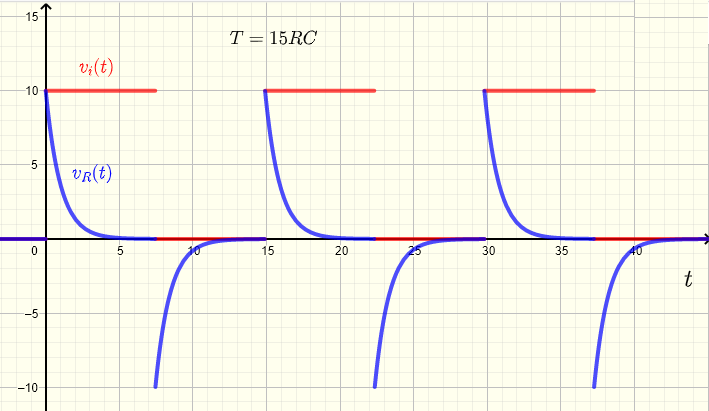Fig.3 - Graphs of the input $v_i(t)$ square wave and the voltage $v_R(t)$ across the resistor for a period T = 15 RC
b) $T = 10 RC = 10$ s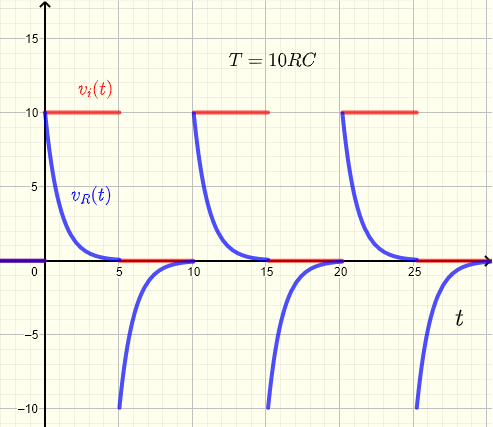Fig.4 - Graphs of the input $v_i(t)$ square wave and the voltage $v_R(t)$ across the resistor for a period T = 10 RC
c) $T = 5 RC = 5$ s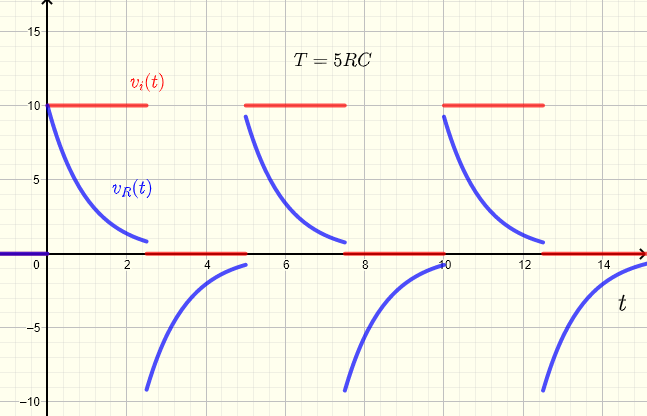Fig.5 - Graphs of the input $v_i(t)$ square wave and the voltage $v_R(t)$ across the resistor for a period T = 5 RC
d) $T = 2 RC = 2$ s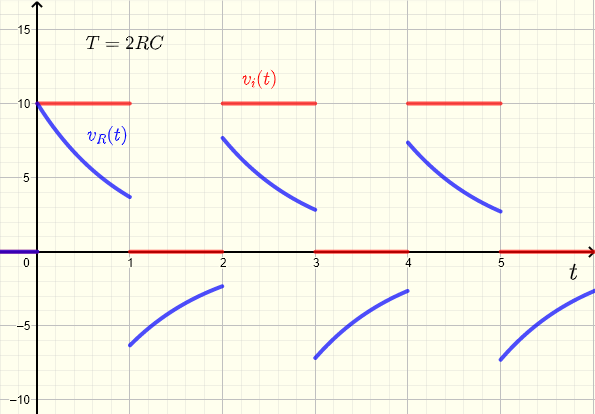Fig.6 - Graphs of the input $v_i(t)$ square wave and the voltage $v_R(t)$ across the resistor for a period T = 2 RC